## Fractran

Fractran is an algorithm applied to a given list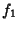,, ...,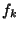of Fractions. Given a starting Integer, the Fractran algorithm proceeds by repeatedly multiplying the integer at a given stage by the first element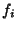given an integer Product. The algorithm terminates when there is no such.

The listwith starting integergenerates a sequence 2, 15, 825, 725, 1925, 2275, 425, 390, 330, 290, 770, ... (Sloane's A007542). Conway (1987) showed that the only other powers of 2 which occur are those with Prime exponent:,,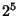,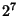, ....

References

Conway, J. H. Unpredictable Iterations.'' In Proc. Number Theory Conf., Boulder, CO, pp. 49-52, 1972.

Conway, J. H. Fractran: A Simple Universal Programming Language for Arithmetic.'' Ch. 2 in Open Problems in Communication and Computation (Ed. T. M. Cover and B. Gopinath). New York: Springer-Verlag, pp. 4-26, 1987.

Sloane, N. J. A. Sequence A007542/M2084 in An On-Line Version of the Encyclopedia of Integer Sequences.'' http://www.research.att.com/~njas/sequences/eisonline.html and Sloane, N. J. A. and Plouffe, S. The Encyclopedia of Integer Sequences. San Diego: Academic Press, 1995.